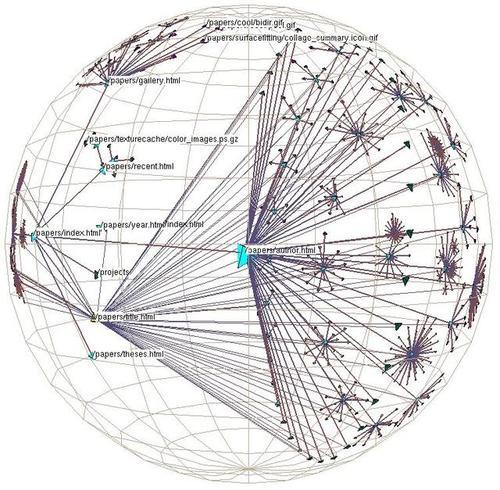# python幂运算是左结合还是右结合_从“位运算”炫技到“操作符”，再到逐步理解“群论”...## 由，再到逐步理解### 下面将举一些具体的位操作运算例子，以辅助大家对各操作符的理解。

### 然后结合上升到的抽象！

0 & 1 = 0

1 & 0 = 0

0 & 0 = 0

1 & 1 = 1

0 | 1 = 1

1 | 0 = 1

0 | 0 = 0

1 | 1 = 1

！0 = 1

！1 = 0

## 异或

1 ^ 0 = 1

0 ^ 1 = 1

0 ^ 0 = 0

1 ^ 1 = 0

### 1.判断一个数的奇偶性（不用％2操作）

n = 3
# if n is even, will be true, else false
def f(n):
return n & 1 == 0

f(n)

False
f(4)

True

### 2.判断一个数是否为2的整数幂

def f(n):
return n & (n-1) == 0

f(4)

True

### 3.不用临时变量交换两个数

def swap(a,b):
a ^= b
b ^= a
a ^= b
return a,b

x,y = 3,4
swap(x,y)

(4, 3)

### 4.找到数组中出现奇数次的元素：

arr = [1,2,1,2,4,3,3]
res = 0
for i in arr:
res ^= i

res
#  任何一个数字异或它自己都等于0。也就是说，如果我们从头到尾依次异或数组中的每一个数字，
#  那么最终的结果刚好是那个只出现奇数次的数字，因为那些出现偶数次的数字全部在异或中抵消掉了。

4

### 5.求两个整数的平均值（结果有小数点时抛弃小数点）

def f(a,b):
return (a+b)>>1

f(5,6)

5

### 6.取两个数的最大值（某些机器上，效率比a>b ? a:b高）

def f(a, b):
return b & ((a-b) >> 31) | a & (~(a-b) >> 31)

f(8,22)

22

### 7.取两个数的最小值（某些机器上，效率比a>b ? b:a高）

def f(a,b):
return a & ((a-b) >> 31) | b & (~(a-b) >> 31)

f(3,4)

3

### 8.判断两个数符号是否相同（0算正号）

def f(x,y):
return (x ^ y) >= 0

f(3,4)

True

### 9.计算2的n次方

def f(n):
return 2<<(n-1)

f(3)

8

### 10.从低位到高位，取某数的第m位

def f(n,m):
return (n >> (m-1))&1

f(8,4)

1

### 11.从低位到高位，将某数的第m位置1

def f(n,m):
return n | (1 <<(m-1))

f(8,2)

10

### 12.取相反数 (注意～是目运算符）
def f(n):
return ~n+1

f(-5)

5

### 13.判断两个数是否相等

def f(x1,x2):
return (x1 ^ x2) == 0

f(3,4)

False

### 14.对n加1，＋＋

def f(n):
return -~n

f(3)

4

### 15.对n减1，－－

def f(n):
return ~-n

f(4)

3

### 16.取绝对值

def f(n):
return (n ^ (n >> 31)) - (n >> 31)

f(-9)

9

## 我们总结一下：

• 任何数和 0 进行 OR 都为自身：x | 0 = x。
• 任何数和 -1 进行 OR 都为 -1：x | -1 = -1。
• 任何数和 0 进行 XOR 都为自身：x ^ 0 = x。
• 任何数和 -1 进行 XOR 都为 ~x：x ^ -1 = ~x。
• 任何数和其自身进行　XOR　都为0：x ^ x = 0。
• 对任何数 x 进行 NOT 操作的结果为 -(x + 1)，~x = -(x+1)。
• <<（左移）该操作数会将第一个操作数向左移动指定的位数。向左被移出的位被丢弃，右侧用0补充。以2的n次方
• >>（符号右移）该操作数会将第一个操作数向右移动指定的位数。向右被移出的位被丢弃，左侧用0补充，最左侧用原符号位填充。以2的n次方

## 群（group）的基本定义：

• （1）封闭性（closure）：对于所有G中a,b，运算a＃b的结果也在G中。
• （2）结合性（Associativity）：对于所有G中的a,b和c，等式 (a#b)#c=a#(b#c)成立。
• （3）单位元（Identity element）：G中存在一个元素e，对于所有G中的元素a，等式 e#a = a#e = a 成立。
• （4）逆元（Inverse element）：对于每个G中的a，存在G中的一个元素b使得 a#b=b#a=e。

## 注意：

• （1）"#"可以是带有任何有意义的操作符，有一些不一定满足交换律，即a#b!=b#a，比如矩阵运算。
• （2）单位元和逆元是唯一的。
• （3）然后群还有　阿贝尔群，循环群，半群，幺半群，子群，群同态，群同构，陪集，商群等概念，数学家总结的一些定理精彩致极！
• （4）你可能会感受到到5次方程的根无解问题原来如此简单
• （5）你可能会看费马小定理变得如此显然，不就是1＋1＝2一样自然的吗？文章同步微信公众号：数学之水，欢迎关注指导

04-15
11-22757
07-21561
07-29963
12-23765
04-281万+
09-25
05-30
06-11
03-08
09-19
06-27

### “相关推荐”对你有帮助么？

•非常没帮助
•没帮助
•一般
•有帮助
•非常有帮助被折叠的  条评论 为什么被折叠?到【灌水乐园】发言点击重新获取扫码支付1.余额是钱包充值的虚拟货币，按照1:1的比例进行支付金额的抵扣。
2.余额无法直接购买下载，可以购买VIP、C币套餐、付费专栏及课程。余额充值